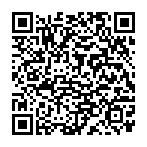## Y6 - Number – Addition, Subtraction, Multiplication and Division - Interactive Quiz

### Related Worksheets

An interactive quiz which test your understanding of all the Number – Addition, Subtraction, Multiplication and Division objectives in the Year 6 curriculum.

Alternatively, you can use the Interactive Maths Quiz which includes objectives from all strands of the Year 6 curriculum.

This quiz tests the following objectives:

• multiply multi-digit numbers up to 4 digits by a two-digit whole number using the formal written method of long multiplication
• divide numbers up to 4 digits by a two-digit whole number using the formal written method of long division, and interpret remainders as whole number remainders, fractions, or by rounding, as appropriate for the context
• divide numbers up to 4 digits by a two-digit number using the formal written method of short division where appropriate, interpreting remainders according to the context
• perform mental calculations, including with mixed operations and large numbers
• identify common factors, common multiples and prime numbers
• use their knowledge of the order of operations to carry out calculations involving the 4 operations
• solve addition and subtraction multi-step problems in contexts, deciding which operations and methods to use and why
• solve problems involving addition, subtraction, multiplication and divisionScan to open this game on a mobile device. Right-click to copy and paste it onto a homework sheet.